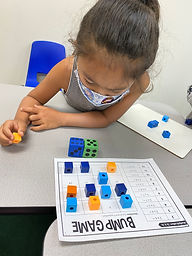Ms. Brianne

Target 1​

Lesson Type:

New

Number Operation

:

Computation

Multiply within 100.

1:

Understand that multiplying numbers makes numbers larger.

2:

Understand that multiplication represents the combination of equal groups of objects.

3:

Understand that in multiplication equations, the first factor represents the number of groups and the second factor represents the quantity in each group.

4:

Understand that multiplication can be represented with arrays, repeated addition, and skip counting.

3rd

Vocabulary:

Multiply, Times, Factor, Product, Equal Groups

Activities:

• Students understood that multiplication involves equal groups, and that that the first factor represents the number of groups, while the second factor tells the number in each group.
• Students used blocks to build equal groups an array board to match multiplication equations.
• Students played a multiplication game to practice solving multiplication problems. They used counting strategies or the array board and blocks to figure out the answers to each multiplication problem.Home Exploration

Guiding Questions:Absent Students:

Target 2

:

1:

Understand that a geometric point is a fixed position in space.

2:

Understand that a line contains an infinite number of points and has no end.

3:

Understand that a line segment is a part of a line, containing two end points (so it has a beginning and an end).

4:

Understand a ray is a combination of a line and a line segment, it has a start point with an endpoint and an arrow which indicates that it extends forever in only one direction.

4th

Vocabulary:

Point, Line, Segment, Ray, Parallel, Perpendicular

Activities:

• Students used playdoh to create different types of lines.
• Students described each type of line that they created, explaining how each one was different from the others.
• Students created their own superhero, incorporating points, lines, line segments, and rays into their design.Home Exploration

Guiding Questions:Target 3

:

Vocabulary:

Activities:Home Exploration